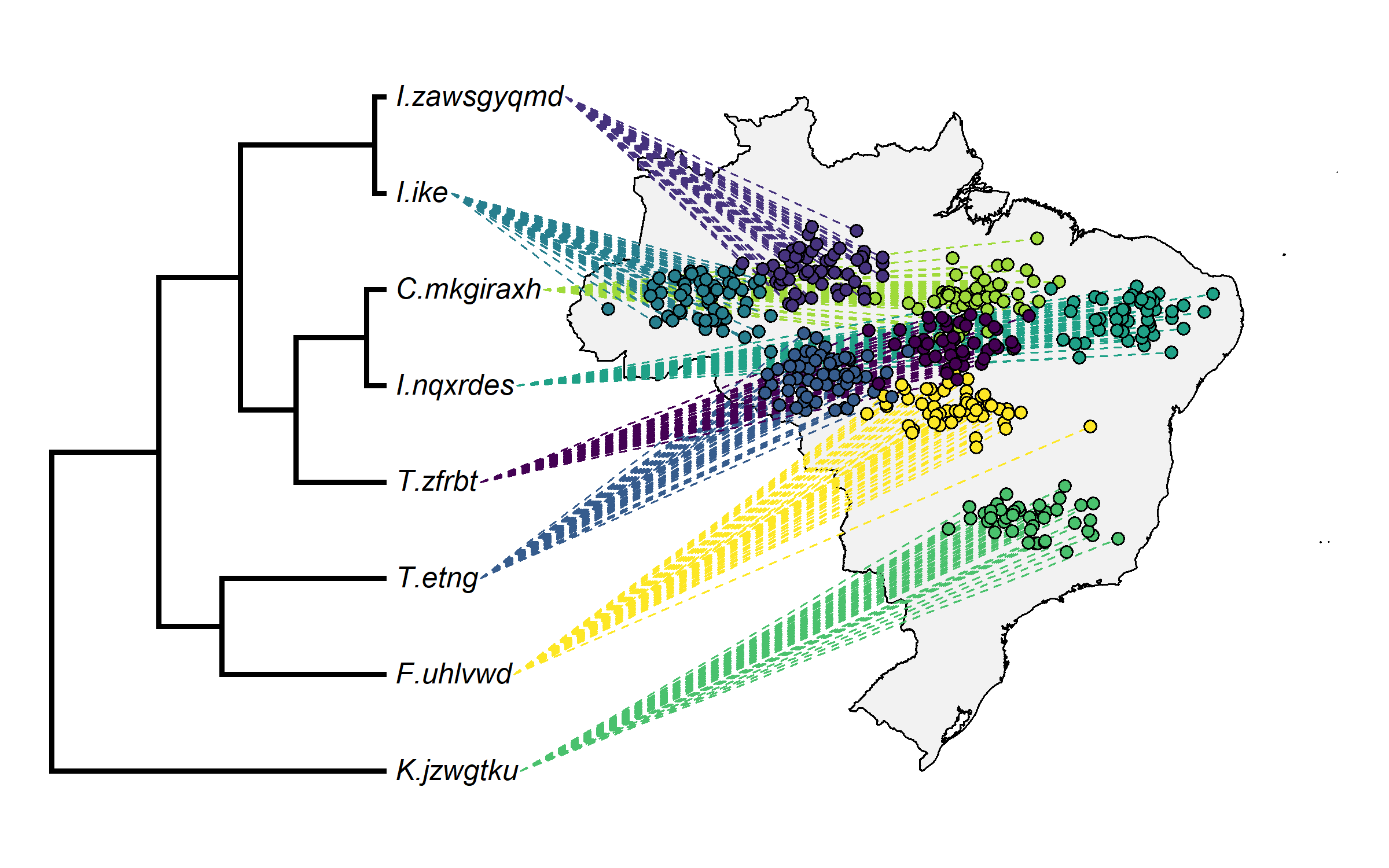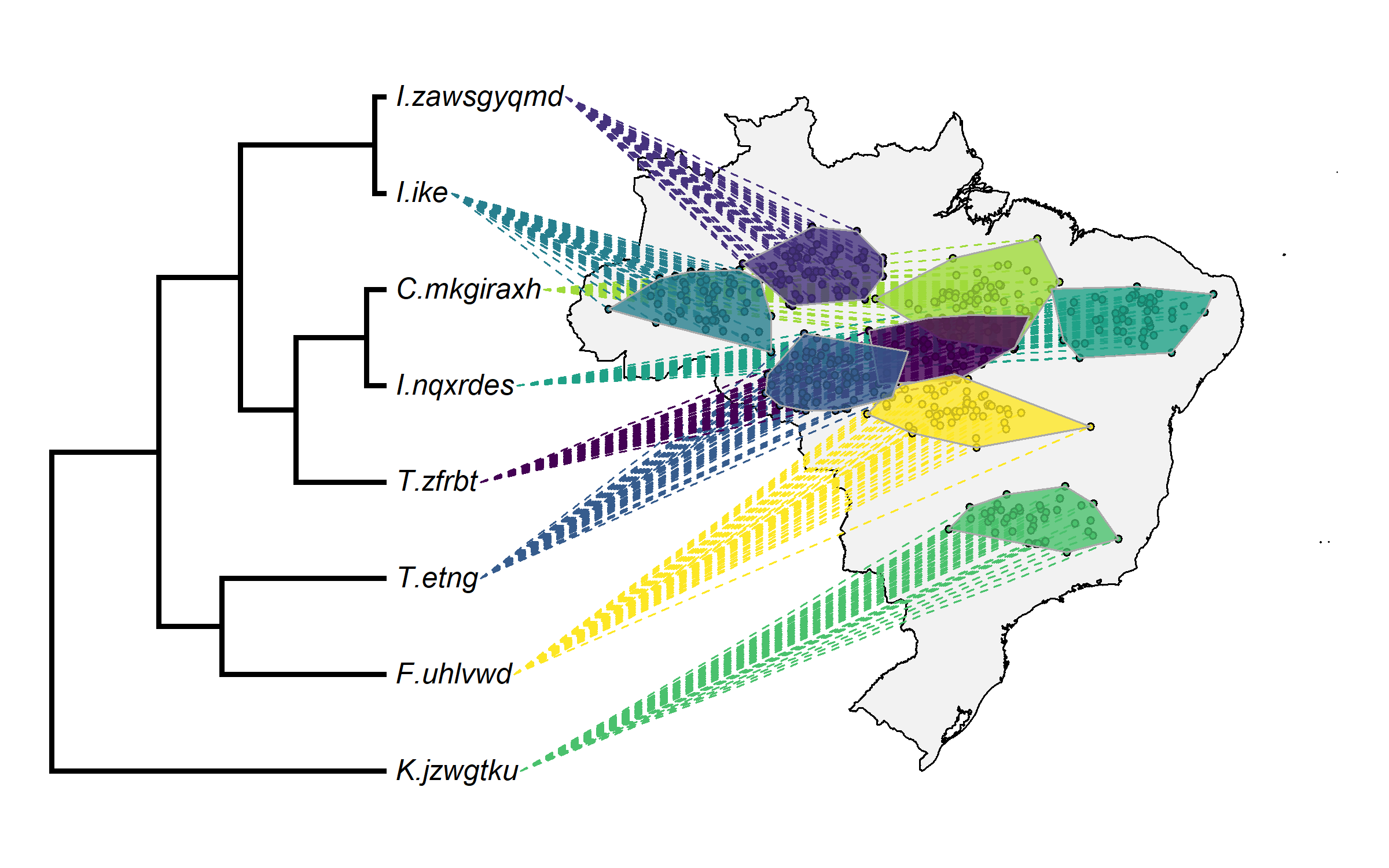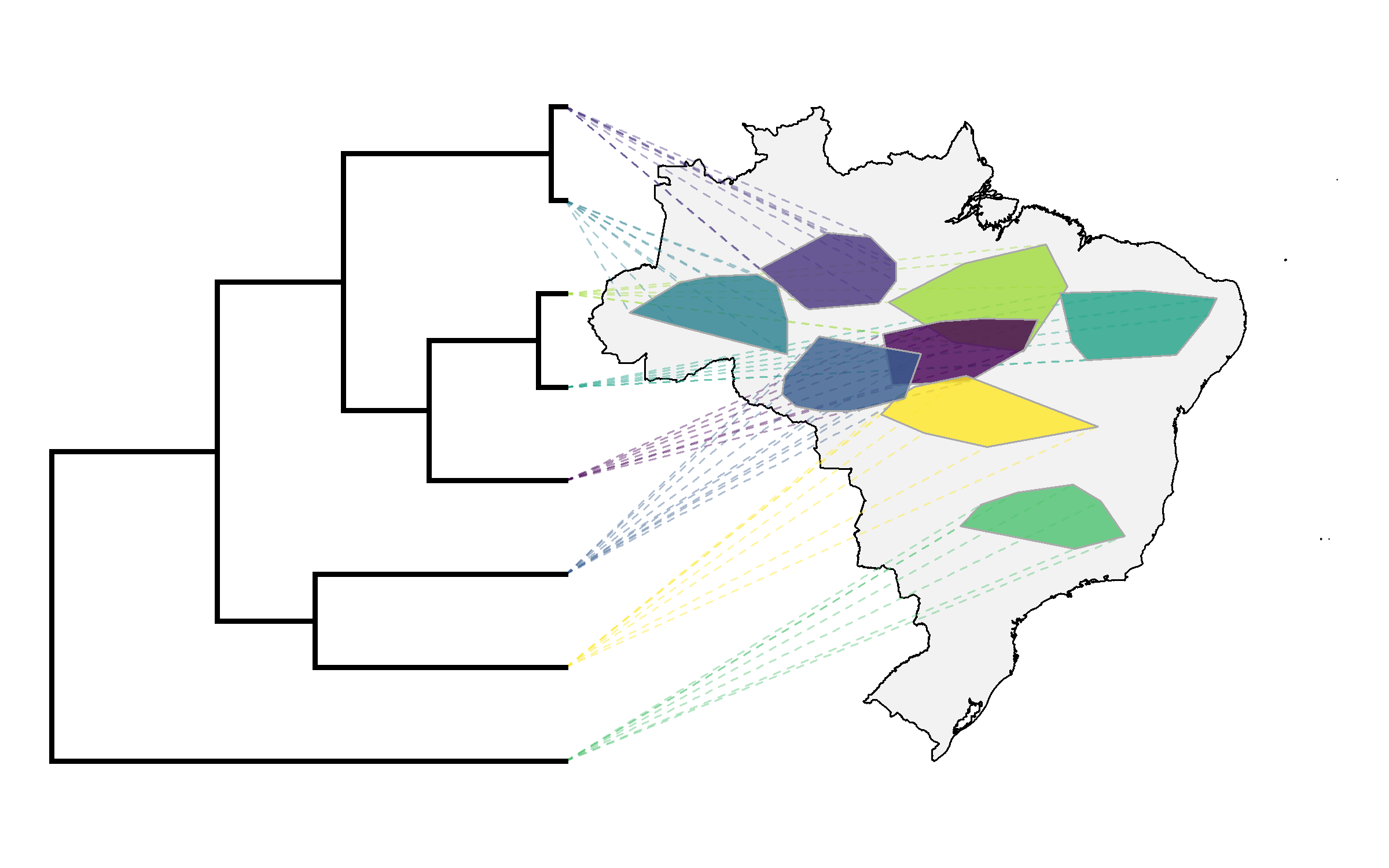## Monday, March 11, 2019

### Projecting a phylogeny onto a geographic map showing species ranges in R

In the past couple of days I have posted a few times (1, 2, 3) about projecting a phylogeny to a geographic map in which we had multiple observations per species.

Today, I thought it might be neat to demonstrate what we can do if we have lots of observations per species and what to project a tree onto estimated range areas for each species. Note that to duplicate this post exactly it is necessary to update phytools from GitHub.

``````library(phytools)
library(mapdata)
Brazil.tree
``````
``````##
## Phylogenetic tree with 8 tips and 7 internal nodes.
##
## Tip labels:
##  I.ike, I.zawsgyqmd, C.mkgiraxh, I.nqxrdes, T.zfrbt, F.uhlvwd, ...
##
## Rooted; includes branch lengths.
``````
``````head(Brazil)
``````
``````##                  lat      long
## C.mkgiraxh -5.923737 -50.30310
## C.mkgiraxh -6.961451 -51.40991
## C.mkgiraxh -6.105770 -51.44966
## C.mkgiraxh -6.133681 -49.31009
## C.mkgiraxh -7.022131 -53.61715
## C.mkgiraxh -4.509163 -49.00638
``````
``````tail(Brazil)
``````
``````##                lat      long
## T.zfrbt  -9.086615 -49.16497
## T.zfrbt  -8.874021 -52.17523
## T.zfrbt  -7.510258 -52.21743
## T.zfrbt  -8.656923 -51.57321
## T.zfrbt -10.740220 -51.95592
## T.zfrbt  -8.993945 -50.38208
``````

Now, let's plot these data just as we did yesterday. Again, I'll use the viridis package (which I just discovered) for colors:

``````library(viridis)
cols<-setNames(sample(viridis(n=Ntip(Brazil.tree))),
Brazil.tree\$tip.label)
``````
``````cols
``````
``````##       I.ike I.zawsgyqmd  C.mkgiraxh   I.nqxrdes     T.zfrbt    F.uhlvwd
## "#277F8EFF" "#46337EFF" "#9FDA3AFF" "#1FA187FF" "#440154FF" "#FDE725FF"
##      T.etng   K.jzwgtku
## "#365C8DFF" "#4AC16DFF"
``````
``````obj<-phylo.to.map(Brazil.tree,Brazil,database="worldHires",
regions="Brazil",plot=FALSE,direction="rightwards")
``````
``````## objective: 24
``````
``````## objective: 12
## objective: 12
``````
``````## objective: 6
``````
``````## objective: 4
``````
``````## objective: 2
## objective: 2
``````
``````plot(obj,direction="rightwards",colors=cols,cex.points=c(0,1),
lwd=c(3,1),ftype="i")
``````That's actually pretty cool looking, but maybe instead of plotting all the points we'd like to plot shapes. One way we could do this is by estimating a convex hull (MCP) for each taxon, and then overlay those at the end:

``````for(i in 1:Ntip(Brazil.tree)){
spr<-Brazil[which(rownames(Brazil)==Brazil.tree\$tip.label[i]),]
mcp<-if(i==1) spr[chull(spr),] else rbind(mcp,spr[chull(spr),])
}
plot(obj,direction="rightwards",colors=cols,cex.points=c(0,0.6),
lwd=c(3,1),ftype="i")
for(i in 1:Ntip(Brazil.tree)){
ii<-which(rownames(mcp)==Brazil.tree\$tip.label[i])
polygon(mcp[ii,2:1],col=make.transparent(cols[Brazil.tree\$tip.label[i]],0.8),
border="darkgrey")
}
``````Or, alternatively, we could plot the shapes without the points:

``````obj<-phylo.to.map(Brazil.tree,mcp,database="worldHires",regions="Brazil",
plot=FALSE,direction="rightwards")
``````
``````## objective: 24
``````
``````## objective: 12
## objective: 12
``````
``````## objective: 6
``````
``````## objective: 4
``````
``````## objective: 2
## objective: 2
``````
``````plot(obj,direction="rightwards",colors=sapply(cols,make.transparent,0.4),
pts=FALSE,ftype="off",cex.points=c(0,0),ftype="off",lwd=c(3,1))
for(i in 1:Ntip(Brazil.tree)){
ii<-which(rownames(mcp)==Brazil.tree\$tip.label[i])
polygon(mcp[ii,2:1],col=make.transparent(cols[Brazil.tree\$tip.label[i]],0.8),
border="darkgrey")
}
``````